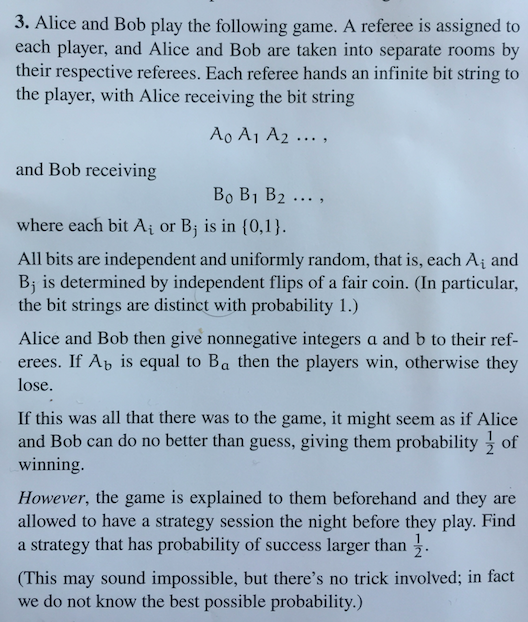# Math Puzzle

Context

Jake and I found ourselves watching New Girl and solving math puzzles last Friday. The. Good. Life.

Problem:Solution

Alice and Bob each pick the index that has the first 1 in their respective arrays.

Intuition

For each permutation length, Alice and Bob nail one case where they necessarily win.

Example

Bob’s array: 001011…

Alice’s array: 011100…

Bob’s guess: 3

Alice’s guess: 2

Note: In this case they do not win, as the second number in Bob’s array (0) is not equal to the third number in Alice’s array (1).

Python script simulation

``````import random

#this creates the randomized bit arrays
def makeBitString(bitlist, length):
for i in range(0, length):
bitlist.append(random.randint(0, 1))

#this is the guessing algorithm. The guess output is the index of your first 1
def guess(bitlist):
index = 0
while (bitlist[index]!=1):
index = index+1
return index+1

#this is one full iteration of the game where each player guesses an index into the other's array. If the corresponding bits are equal the players win, otherwise they lose
def match(len):
blistA = []
blistB = []
makeBitString(blistA, len)
makeBitString(blistB, len)
guessA = guess(blistA)
guessB = guess(blistB)
if (blistA[guessB-1] == blistB[guessA-1]):
return 1
else:
return 0

#simulates a number of matches w/ array length len
def simulate(matches, len):
wins = 0
losses = 0
for i in range(0, matches):
if (match(len) == 1):
wins = wins + 1
else:
losses = losses + 1
print "this is how many you won"
print wins
print "this is how many you lost"
print losses

simulate(10000, 20)

``````